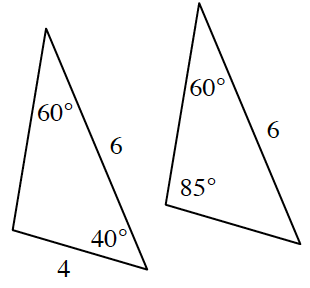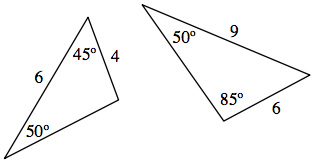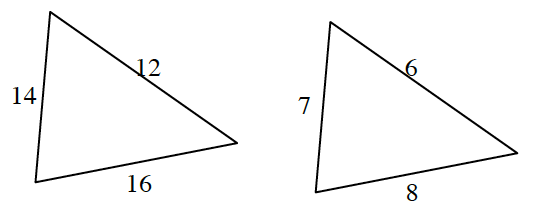### Home > INT2 > Chapter 2 > Lesson 2.3.1 > Problem2-84

2-84.

Determine which of the following pairs of triangles are similar. Justify your answer.

1.Find the missing angle measure for each triangle to see if $AA\sim$ applies.

The triangles are not similar because they have different angle measures.

1.Find the missing angle measure for each triangle to see if $AA\sim$ applies.

1.Write a ratio for each pair of sides. If the triangles are similar, all three ratios should be equal.

Is this true?

$\frac{14}{7}=\frac{12}{6}=\frac{16}{8}$